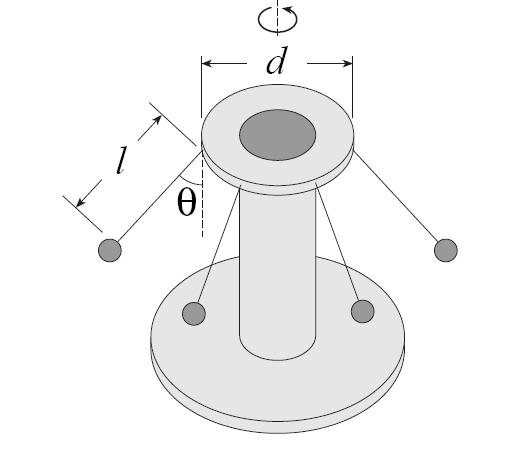# An amusement park ride question, picture included

## Homework StatementAn amusement park ride consists of a rotating circular platform 8.07m in diameter from which 10kg seats are suspended at the end of 2.87m massless chains. When the system rotates, it makes an angle of 37.3° with the vertical.
a) What is the speed of each seat?
b) If a child of mass 45.3kg sits in a seat what is the tension in the chain for the same angle?

m=55.3kg (mass of the seat + the mass of the child)
Vt=5.56565m/s (calculated from part A, confirmed as correct)
Ѳ=37.3° (listed)
g=9.8m/s2 (listed)
d=8.07m (listed)
l=2.87m (listed)
r=5.77419m (calculated from the length of the chain and the diameter of the circular platform, d/2+lsinѲ, which is its horizontal distance from the center of rotation, where d is the diameter of the platform and l is the length of the chain)

## Homework Equations

ƩFx=0
ƩFy=0
Fc=$$\frac{Vt^2m}{r}$$
Fg=mg

## The Attempt at a Solution

I have the answer to A, and that part is verified as correct already. B is what I'm wondering about.

So, this swing is rotating in the z dimension, but in the x and y directions it is in equilibrium which means that the sum of the forces in the x direction and the y direction must be equal to 0.

ƩFx=0
ƩFy=0

Then the forces in the x are the horizontal tension in the cable in the x and the centrifugal (equal and opposite to the centripetal), and the forces in the y are the vertical tension in the cable and the force of gravity.

ƩFx=Tx-Fc
ƩFy=Ty-Fg

Since they're both equal to 0, they can be set equal to each other.
Tx-Fc=Ty-Fg

I rewrote these based on their definitions.

Tsin(Ѳ)-$$\frac{Vt^2m}{r}$$=Tcos(Ѳ)-mg

Solve symbolically:
T=$$\frac{m}{sin\Theta-cos\Theta}$$$$\frac{Vt^2}{r}$$ -g

When I put all that together, I get T=681.28N. I have one more guess left for this problem so I need to be sure it's right beforehand. Can someone verify if this logic is correct please?

Andrew Mason
Homework Helper
You are making it more complicated than necessary. All you have to do is determine Ty. You know the angle, so you can determine T from that.

AM

It doesn't ask for the vertical component of the tension. It asks for the tension overall.

Andrew Mason
Homework Helper
It doesn't ask for the vertical component of the tension. It asks for the tension overall.
Yes. But with the vertical component of T and the angle you can easily find T.

AM

So...like Ty/cos$$\Theta$$?

Andrew Mason
Homework Helper
So...like Ty/cos$$\Theta$$?
That's right.

AM

Wow. I can't believe I didn't think of that. And it equals the same thing I got but with so much less work. Thank you very much.

Andrew Mason
Homework Helper
You are welcome. And welcome to PF by the way.

AM

Wait...okay so I have another question.

So Tcos$$\Theta$$=mg. And when I solved for T, it gave me the same answer of 681.28N as the supercomplicated one. But my other equation was Tsin$$\Theta$$=Vt^2m/r. When I solved that one, I got T=489.555N. It should be the same, right? So what's different about it? Am I missing a force in the x direction that I should take into consideration?

Andrew Mason
Homework Helper
Wait...okay so I have another question.

So Tcos$$\Theta$$=mg. And when I solved for T, it gave me the same answer of 681.28N as the supercomplicated one. But my other equation was Tsin$$\Theta$$=Vt^2m/r. When I solved that one, I got T=489.555N. It should be the same, right? So what's different about it? Am I missing a force in the x direction that I should take into consideration?
Since $T\cos\theta = mg$ is always true, the tension is easily determined. It is simply a matter of relating the x component of tension to the centripetal force: $T\sin\theta = mv^2/r = m\omega^2r$. It is a bit tricky because r is a function of $\theta$. I am not sure what you were using for r but your formula is correct.

AM

Andrew Mason
Homework Helper
As a follow up to this, I get 6.56 m/sec as a value for v (tangential speed of the chairs) not 5.65 m/sec.

$$\frac{mg}{cos\theta} = \frac{mv^2}{r}$$

$$v = \sqrt{g\tan\theta(L\sin\theta + d/2)}$$

$$v = \sqrt{9.8*.762*(2.87*.606 + 4.035)$$

$$v = \sqrt{43.12} = 6.56 m/sec$$

AM

hey. can i ask something about this question. how to get the V for the part (a). is it something to do with the horizontal and vertical forces which involve sin and cos? i am still not able to get it :(